# Placing Three 5-Digit Numbers in Descending Order

In this worksheet, students place three numbers in descending order of size.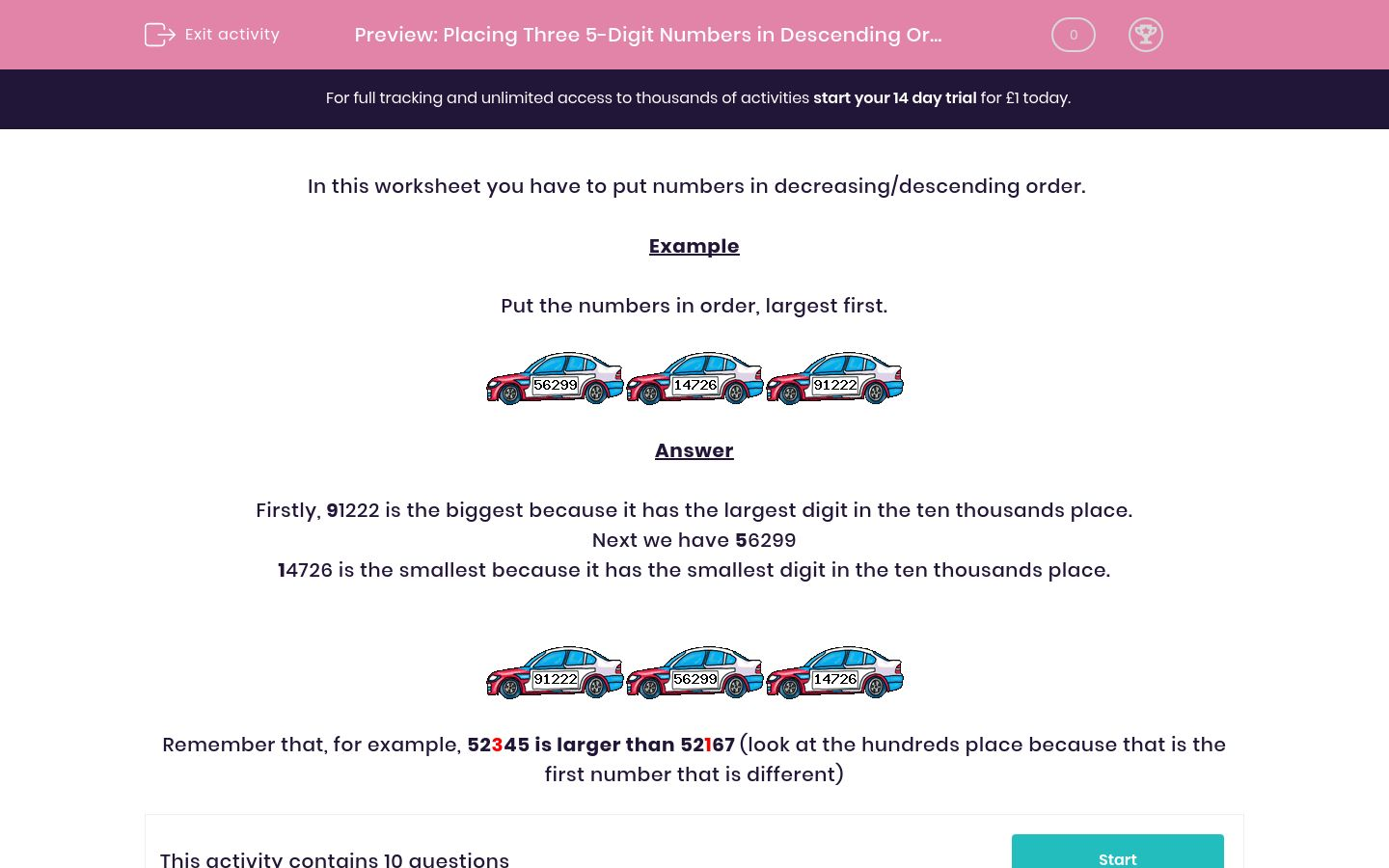Key stage:  KS 2

Curriculum topic:   Maths and Numerical Reasoning

Curriculum subtopic:   Place Value

Difficulty level:### QUESTION 1 of 10

In this worksheet you have to put numbers in decreasing/descending order.

Example

Put the numbers in order, largest first.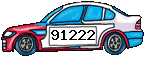Firstly, 91222 is the biggest because it has the largest digit in the ten thousands place.

Next we have 56299

14726 is the smallest because it has the smallest digit in the ten thousands place.Remember that, for example, 52345 is larger than 52167 (look at the hundreds place because that is the first number that is different)

Put the numbers in order, largest first.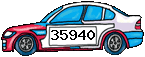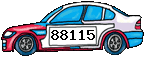1 2 3 14221 35940 88115

Put the numbers in order, largest first.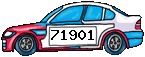1 2 3 27513 71901 90895

Put the numbers in order starting with the largest.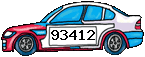1 2 3 89799 64393 93412

Put the numbers in order, largest first.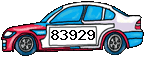1 2 3 79733 83929 40476

Put the numbers in descending order.1 2 3 68738 28567 39851

Put the numbers in decreasing order.1 2 3 57024 98670 12160

Put the numbers in descending order.1 2 3 92020 54035 31576

Put the numbers in order, largest first.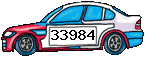1 2 3 33984 83949 50615

Put the numbers in order, largest first.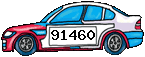1 2 3 48284 41708 91460

Put the numbers in descending order.1 2 3 91977 40133 46071
• Question 1

Put the numbers in order, largest first.1 2 3 14221 35940 88115
• Question 2

Put the numbers in order, largest first.1 2 3 27513 71901 90895
• Question 3

Put the numbers in order starting with the largest.1 2 3 89799 64393 93412
• Question 4

Put the numbers in order, largest first.1 2 3 79733 83929 40476
• Question 5

Put the numbers in descending order.1 2 3 68738 28567 39851
• Question 6

Put the numbers in decreasing order.1 2 3 57024 98670 12160
• Question 7

Put the numbers in descending order.1 2 3 92020 54035 31576
• Question 8

Put the numbers in order, largest first.1 2 3 33984 83949 50615
• Question 9

Put the numbers in order, largest first.1 2 3 48284 41708 91460
• Question 10

Put the numbers in descending order.1 2 3 91977 40133 46071
---- OR ----

Sign up for a £1 trial so you can track and measure your child's progress on this activity.

### What is EdPlace?

We're your National Curriculum aligned online education content provider helping each child succeed in English, maths and science from year 1 to GCSE. With an EdPlace account you’ll be able to track and measure progress, helping each child achieve their best. We build confidence and attainment by personalising each child’s learning at a level that suits them.

Get started